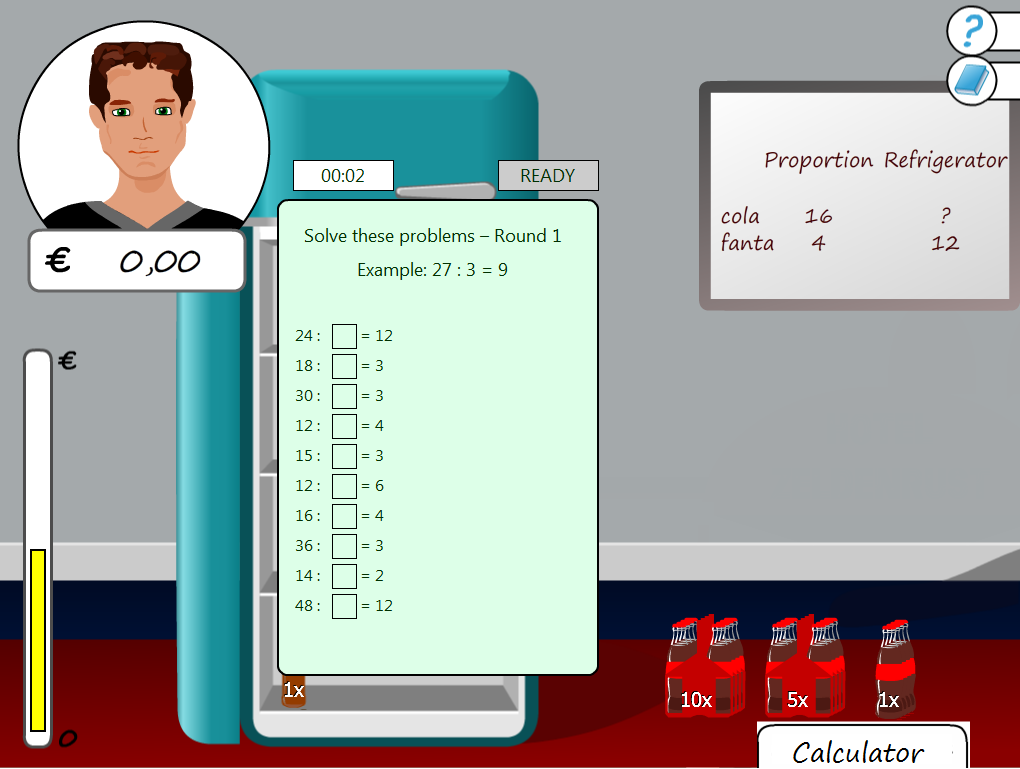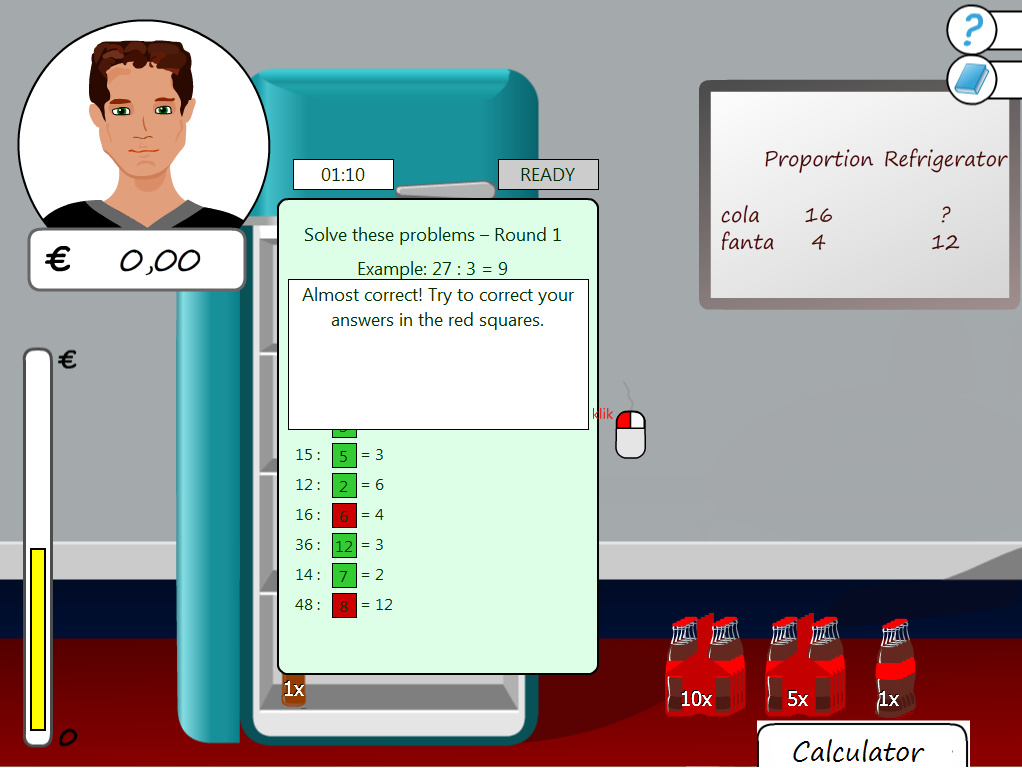Solving proportional reasoning problems supposes specific well-trained mathematical skills such as good mastery of the multiplication tables. In the part-task practice version the player receives exercises to practice these skills. The player receives these exercises in the first three levels of each subgame before the subgame starts. A presentation of exercises involves two rounds with each 10 exercises. After each round the player has the opportunity to correct the exercises that were wrong.##### First round of part-task practice for multiplication exercises